# Exercise / Fitness

Grid List
\$7.15
Increase value Decrease value
\$34.00
Increase value Decrease value
\$72.55
Increase value Decrease value
\$61.86
Increase value Decrease value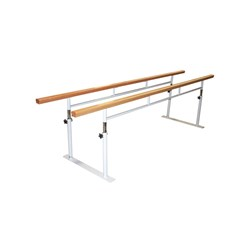3 Meter Folding Walking Rails
\$1418.04
Increase value Decrease value
\$19.99
Increase value Decrease value
\$19.99
Increase value Decrease value
\$12.99
Increase value Decrease value
\$17.00
Increase value Decrease value
\$19.99
Increase value Decrease value
\$29.99
Increase value Decrease value
\$50.00
Increase value Decrease value
\$38.50
Increase value Decrease value
\$34.99
Increase value Decrease value
\$34.99
Increase value Decrease value
\$19.99
Increase value Decrease value
\$39.99
Increase value Decrease value
\$99.99
Increase value Decrease value
\$39.99
Increase value Decrease value
PTP Ab Sculpt
In Stock
\$50.00
Increase value Decrease value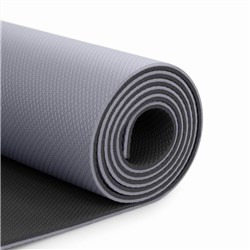\$44.00
Increase value Decrease value
\$59.99
Increase value Decrease value
BAHE Yoga Wheel
In Stock
\$69.99
Increase value Decrease value
\$19.99
Increase value Decrease value
\$34.99
Increase value Decrease value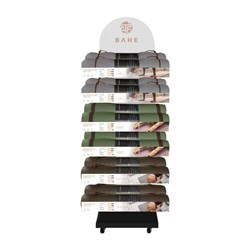\$499.00
Increase value Decrease value
\$299.00
Increase value Decrease value
\$32.00
Increase value Decrease value
\$340.00
Increase value Decrease value
Alpha Sport Balance Beam Long (195cm)
\$218.90
Increase value Decrease value
Practitioner Tubing 30m [Silver-Super-Strong]
\$115.60
Increase value Decrease value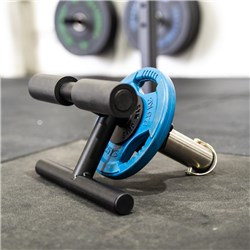The TIB BAR
\$199.00
Increase value Decrease value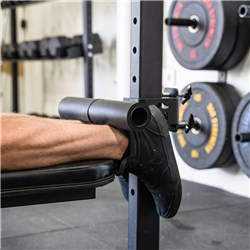The Nordic Bar
Backorder
\$239.00
Increase value Decrease value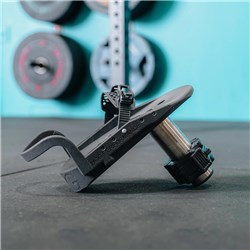\$149.00
Increase value Decrease value\$130.00
Increase value Decrease value
\$16.25
Increase value Decrease value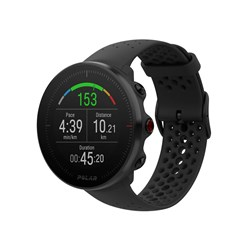\$346.50
Increase value Decrease value
\$279.20
Increase value Decrease value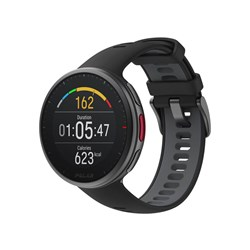\$616.00
Increase value Decrease value
\$111.20
Increase value Decrease value
\$39.95
Increase value Decrease value
\$49.96
Increase value Decrease value
\$154.00
Increase value Decrease value
\$39.95
Increase value Decrease value
\$169.99
Increase value Decrease value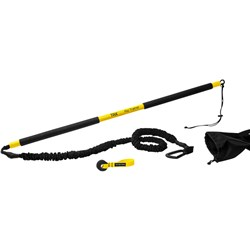TRX Rip Trainer
In Stock
\$275.00
Increase value Decrease value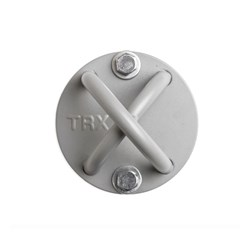\$53.49
Increase value Decrease value
\$499.00
Increase value Decrease value
Grid List
##### Products to compare:
Comparing Products